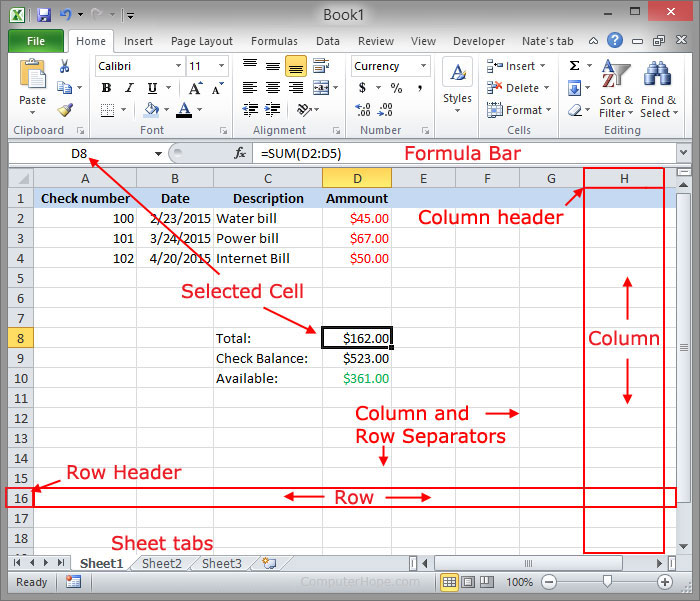Welcome to Class !!

In today’s Computer Science class, We will be discussing Spreadsheets. We hope you enjoy the class!

Spreadsheets are like lined paper with columns. The sheet itself comprises of columns (vertical) and rows (horizontal) which are in turn, made up of cells. A spreadsheet is the computer equivalent of a paper ledger sheet.A spreadsheet can be defined as the combination of rows and columns used in performing arithmetic calculations. It was first constructed in 1979 and has developed into one of the most widely used software products during the 1980s. It is widely used in a diverse range of disciplines such as mathematics, engineering, science, medicine, the arts, social science, accounting and education.

An electronic spreadsheet is an application package used mostly in accounting, statistics, financial and inventory control.

Spreadsheet package is a software that allows the use of numerical data and thereby performing automatic calculations on numbers contained in a table. Spreadsheet packages are used to handle accounting problems, financial analysis, budgeting, charting and graphing, scientific applications, inventory, statistical data, etc.

In a spreadsheet, as you enter the numbers, the computer puts them on to the screen in neat columns. You can tell the program to compute the totals, subtotals, percentages and show them on the screen faster than your eye can blink.

1. Lotus 1-2-3
2. SuperCalc
3. Visicalc
4. Microsoft Excel
5. Corel Quattro.
6. StatView

EVALUATION

2. Mention THREE types of spreadsheet applications.

Microsoft Excel is preferable to the other earlier packages because:

• It is user friendly.
• It is flexible.1. Spreadsheets are used for calculations of large figures
2. They are used to enter text, numerical values and formulas into electronic worksheets.
3. We use spreadsheets to view data in various formats (pie charts, line graphs and bar graphs).
4. They can be used for collection and records analysis.
5. They are used in preparing budgets.
6. They are used for preparing examination results.

GENERAL EVALUATION

1. List THREE uses of spreadsheet applications.
2. Why is Ms excel more preferable than other spreadsheet programs.
4. Apart from Microsoft excel, list TWO other types.

READING ASSIGNMENT: SIMPLE GUIDE TO COMPUTER PAGE 45

WEEKEND ASSIGNMENT

1. Which of these programs can calculate large numbers? A. Ms word B. Ms access B. spreadsheet programs. D. all
2. An analysis can be made with which of these applications. A. Ms word B. Ms access B. spreadsheet programs. D. all
3. All except one is not an example of spreadsheet programs. A. Lotus 1-2-3  B. Supercal C. Visicalc D. Ms Word.
4. …………….contains rows and columns. A. Spreadsheet B. Workbook C. Ms word. D. All
5. Preparing examination results can easily be computed with………… A. spreadsheet B. workbook C. Ms word. D. all

THEORY

We have come to the end of this class. We do hope you enjoyed the class?

Should you have any further question, feel free to ask in the comment section below and trust us to respond as soon as possible.

In our next class, we will be talking about Spreadsheet Packages. We are very much eager to meet you there.